# ISEE Middle Level Quantitative : Numbers and Operations

## Example Questions

1 2 47 48 49 50 51 52 53 55 Next →

### Example Question #81 : Use Parentheses, Brackets, Or Braces In Numerical Expressions And Evaluate These Expressions: Ccss.Math.Content.5.Oa.A.1

Solve: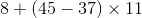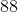Explanation:When solving this problem, remember order of operations PEMDAS. The parentheses come first, followed by the multiplication, and then the addition.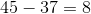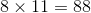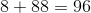### Example Question #82 : Common Core Math: Grade 5

Solve: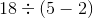Explanation:When solving this problem, remember order of operations PEMDAS. The parentheses come first followed by the division.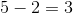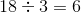### Example Question #81 : Common Core Math: Grade 5

Solve: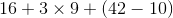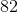Explanation:When solving this problem, remember order of operations PEMDAS. The parentheses come first, followed by the multiplication, and then addition.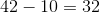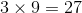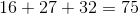1 2 47 48 49 50 51 52 53 55 Next →

### All ISEE Middle Level Quantitative Resources